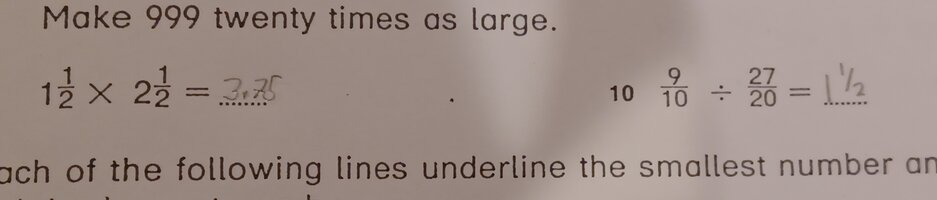# Multiplying fractions: 1 1/2 + 1 1/2, and 9/10 divided by 27/20

#### LiseyDot05

##### New member
Me again.I hope I haven't posted this in the wrong section, apologies if I have!

I would just appreciate some guidance on the following math problems and whether he got them right and if he solved them in the easiest/best way possible.

The first is: 1 ½ x 2 ½
For this my son worked it out by turning them into
³/² x ⁵/² = ¹⁵/⁴. Turning that into 3.75 or 3 ¾ for the answer.

The second problem is: ⁹/¹⁰ ÷ ²⁷/²⁰
For this my son worked it out by
27 ÷ 9 = 3 & 20 ÷ 10 = 2 giving him ³/² which he converted to 1 ½ as the answer.

I have probably explained that terribly but I hope someone can decipher it.#### Dr.Peterson

##### Elite Member
Me again.I hope I haven't posted this in the wrong section, apologies if I have!

I would just appreciate some guidance on the following math problems and whether he got them right and if he solved them in the easiest/best way possible.

The first is: 1 ½ x 2 ½
For this my son worked it out by turning them into
³/² x ⁵/² = ¹⁵/⁴. Turning that into 3.75 or 3 ¾ for the answer.

The second problem is: ⁹/¹⁰ ÷ ²⁷/²⁰
For this my son worked it out by
27 ÷ 9 = 3 & 20 ÷ 10 = 2 giving him ³/² which he converted to 1 ½ as the answer.

I have probably explained that terribly but I hope someone can decipher it.

View attachment 36340
The first is correct, and done in what is probably the easiest way in terms of fractions; if the answer is expected to be a decimal, he could have done the work in decimal form, [imath]1.5\times2.5[/imath], perhaps a little more easily.

For the second, he divided backwards, apparently just because that was easier. A method like this can work (dividing 9 by 27 and 10 by 20), but it sounds like he may have just made up the method rather than having a reason to be sure it would be correct.

A clue that the answer is wrong is that dividing a smaller number by a larger number should give an answer less than 1!

The usual method is to change the division into a multiplication: [imath]\dfrac{9}{10}\times\dfrac{20}{27}[/imath], and do that any way you like.

Also, just in case, I have to ask: are you sure the "10" is not part of the problem? I don't see any other problem numbers here, so I would have taken it as [imath]10\dfrac{9}{10}\div\dfrac{27}{20}[/imath].

•stapel

#### lev888

##### Elite Member
Me again.I hope I haven't posted this in the wrong section, apologies if I have!

I would just appreciate some guidance on the following math problems and whether he got them right and if he solved them in the easiest/best way possible.

The first is: 1 ½ x 2 ½
For this my son worked it out by turning them into
³/² x ⁵/² = ¹⁵/⁴. Turning that into 3.75 or 3 ¾ for the answer.

The second problem is: ⁹/¹⁰ ÷ ²⁷/²⁰
For this my son worked it out by
27 ÷ 9 = 3 & 20 ÷ 10 = 2 giving him ³/² which he converted to 1 ½ as the answer.

I have probably explained that terribly but I hope someone can decipher it.

View attachment 36340
For multiplication, double-check in what form the answer should be written (fraction, decimal, mixed).
Division: it looks like your son needs to review the fraction division chapter. It should mention that dividing by a fraction is equivalent to multiplying by its reciprocal. See Dr. Peterson's post.

•stapel

#### LiseyDot05

##### New member
I just had to check the booklet & the problem number is 10. It's just the awkward way I've cropped it to avoid confusion.•Dr.Peterson

#### Steven G

##### Elite Member
(9/10)÷(27/10).
Whether 9/10 is more than 1, less that 1 or equal to 1, since we are dividing by 27/10 which is more than1 (actually it is more than 2) the result will be LESS THAN 9/10. Your son's answer of 1 1/2 is larger than 9/10! Your son MUST think about whether any answer he comes up with is a logical answer.
He should think the following. I have less that $1 (since 9/10 <1) and I am sharing this money with more than 2 people (27/10 >2) so can each person get$1 1/2? Of course if you have less that $1 and share it equally with more than people, then it is impossible for everyone to get$1-1/2.

I make more mistakes than I prefer to admit to, but I catch almost all of them by thinking if my answer makes any sense.

•khansaheb and stapel

#### Steven G

##### Elite Member
I was thinking that your son should know the distributive law and should know that 1 1/2 = 1 + 1/2 & that 2 1/2 = 2+1/2.

So 1 1/2 x 2 1/2 = (1 + 1/2)x(2 + 1/2) = 1x2 + 1x1/2 + 1/2x2 + 1/2x1/2 = 2 + 1/2 + 1 + 1/4 = 3 + 3/4 = 3 3/4 or 15/4 or 3.75

•LiseyDot05 and stapel